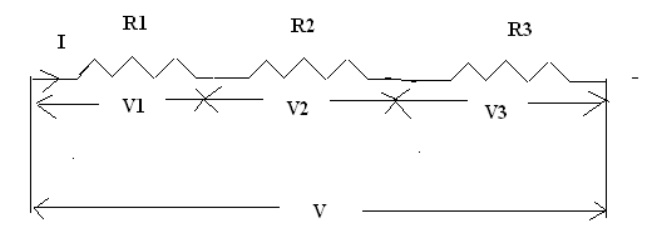Home | | Basic Electrical and Electronics Engineering | Steady State Solution of DC Circuits and Problems based on ohm‚Äôs law

# Steady State Solution of DC Circuits and Problems based on ohm‚Äôs law

Resistance in parallel connection: Resistance in parallel connection: Steady State Solution of DC Circuits and Problems based on ohm‚Äôs law

Steady State Solution of DC Circuits:

Resistance in series connection:The resistors R1, R2, R3 are connected in series across the supply voltage ‚ÄúV‚Äù. The total current flowing through the circuit is denoted as ‚ÄúI‚Äù. The voltage across the resistor R1, R2 and R3 is V1, V2, and V3 respectively.

V1 = I*R1 (as per ohms law)

V2= I*R2

V3 = I*R3

V = V1+V2+V3

= IR1+IR2+IR3

= (R1+R2+R3) I IR = (R1+R2+R3) I

R = R1+R2+R3

Resistance in parallel connection:The resistors R1, R2, R3 are connected in parallel across the supply voltage ‚ÄúV‚Äù. The total current flowing through the circuit is denoted as ‚ÄúI‚Äù. The current flowing through the resistor

R1, R2 and R3 is I1, I2, and I3 respectively.

I = V / R (as per ohms law)

I 1 = V1 / R1

I2 = V2 / R2

I3 = V3 / R3

V1 = V2 = V3 = V

From the above diagram

I  = I1+I2+I3

= V1 / R1 + V2 / R2 + V3 / R3

= V / R1+ V/R2 +V/R3

I  = V (1/R1 +1/R2 +1/R3)

V / R = V (1/R1 +1/R2 +1/R3)

1/R = 1/R1 +1/R2 +1/R3

Problems based on ohm‚Äôs law

Problem 1:

A current of 0.5 A is flowing through the resistance of 10‚Ñ¶.Find the potential difference between its ends.

Given data:

Current  I= 0.5A.

Resistance R=1‚Ñ¶

T o f i n d

Potential difference V = ?

Formula used:

V = IR

Solution:

V = 0.5 √ó 10 = 5V.

Result :

The potential difference between its ends = 5 V

Problem :2

A supply voltage of 220V is applied to a 100 ‚Ñ¶ resistor. Find the current flowing through

it.

Given data

Voltage V =  220V

Resistance R   = 100‚Ñ¶

To find:

Current I = ?

Formula used:

Current I = V / R

Solution:

Current I = 220/100

= 2.2 A

Result:

The current flowing through the resistor = 2.2 A

Problem : 3

Calculate the resistance of the conductor if a current of 2A flows through it when the potential difference across its ends is 6V.

Given data

Current I = 2A

Voltage V = 6V

To find:

Resistance R = ?

Formula used:

Resistance R = V / I

Solution:

Resistance R = 6 / 2

Result:

The value of resistance R = 3Œ©

Problem: 4

Calculate the current and resistance of a 100 W,  200V electric bulb.

Given data:

Power P = 100W

Voltage V = 200V

To find:

Current I =?

Resistance R =?

Formula used:

Power P = V *I

Current I = P / V

Resistance R = V / I

Solution:

Current I = P / V

= 100 / 200

= 0.5 A Resistance R = V / I

= 200 / 0.2

Result:

The value of the current I = 0.5 A

The value of the Resistance R = 400 Œ©

Problem: 5

A circuit is made of 0.4 ‚Ñ¶ wire, a 150‚Ñ¶ bulb and a 120‚Ñ¶ rheostat connected in series. Determine the total resistance of the circuit.

Given data:

Resistance of the wire = 0.4‚Ñ¶

Resistance of bulb       = 1 5 0 ‚Ñ¶

Resistance of rheostat =        120‚Ñ¶

To find:

The total resistance of the circuit R T =?

Formula used:

The total resistance of the circuit R T = R1+R2+R3

Solution:

Total resistance ,R               =  0.4 + 150 +120

= 270.4‚Ñ¶

Result:

The total resistance of the circuit R T = 270.4 Œ©

Problem 6:

Three resistances of values 2‚Ñ¶, 3‚Ñ¶ and 5‚Ñ¶ are connected in series across 20 V, D.C  supply

.Calculate (a) equivalent resistance of the circuit (b) the total current of the circuit (c) the voltage drop across each resistor and (d) the power dissipated in each resistor.

Given data:

V = 20V

To find:

R T =?

I T =?

V1, V2, V3 =?

P1, P2, P3 =?

Formula used:

RT = R1+R2+R3 (series connection)

IT = VT / RT

V1 = R1*I1

V2= R2*I2

V3 = R3*I3

P1=V1*I1

P2=V2*I2

P3=V3*I3

Solution:

RT = R1+R2+R3 = 2+3+5

IT = VT / RT = 20 / 10

IT = 2 A

In series connection I1 = I2 = I3 = IT = 2A

V1 = I1*R1 = 2*2

V1 = 4 V

V2 = I2*R2 = 2*3

V2 = 6 V

V3 = I3*R3 = 5*2

V3 = 10V

P1 = V1*I1

= 4*2

P1 = 8W

P2 = V2*I2

= 6*2

P2 = 12W

P3 = V3*I3 = 10*2

P3 = 20W

Result:

(a). Equivalent resistance of the circuit RT = 10Œ©

(b). The total current of the  circuit IT = 2A

(c). Voltage drop across each resistor V1 = 4 V, V2 = 6 V, V3 = 10V

(d). The power dissipated in each resistor P1 = 8W, P2 = 12W, P3 = 20W

Study Material, Lecturing Notes, Assignment, Reference, Wiki description explanation, brief detail
Basic Electrical and electronics : Electric Circuits and Measurements : Steady State Solution of DC Circuits and Problems based on ohm‚Äôs law |